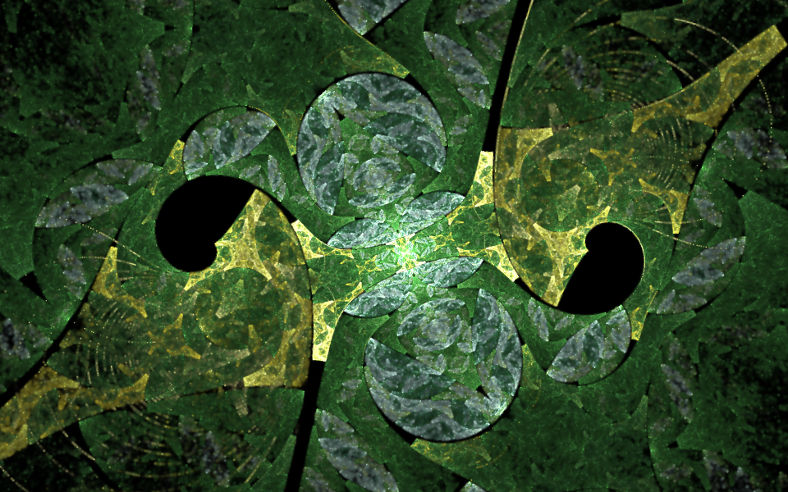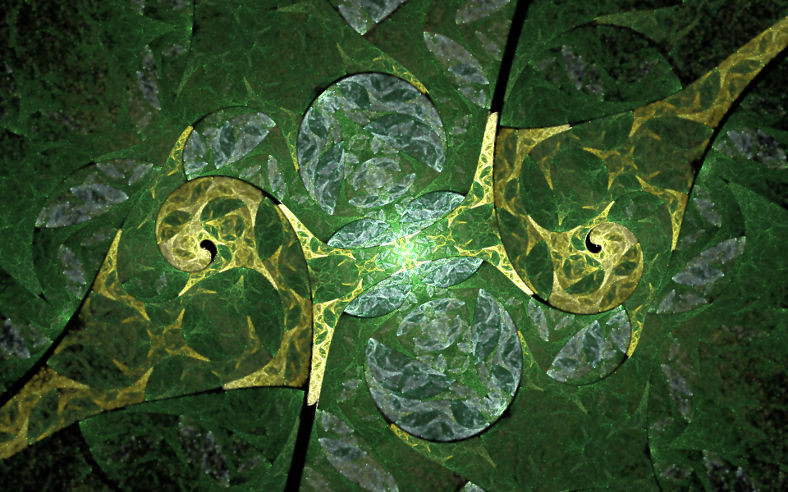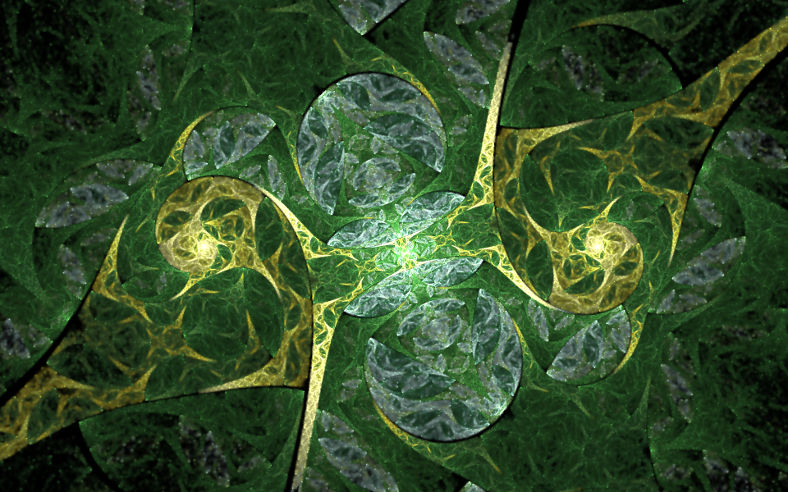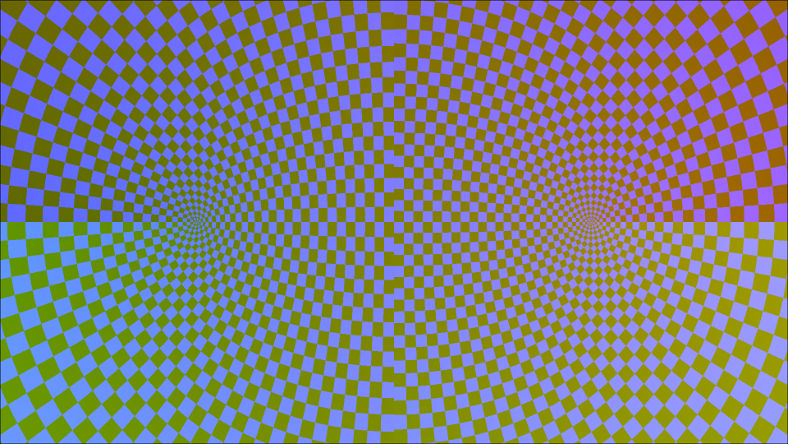# mathr / blog / #

## A more accurate elliptic variation

On the fractal chats Discord server, it was discussed that the "elliptic" variation in fractal flame renderers suffered from precision problems. So I set about trying to fix them. The test parameters are here: elliptic-precision-problems.flame. It looks like this:The black holes are the problem. Actually it turns out that the main cause of the hole was the addition of an epsilon to prevent division by zero in the "spherical variation", removing that gives this image, still with small black holes in the spirals:The original code for the flam3 implementation of the elliptic variation is:

void var62_elliptic (flam3_iter_helper *f, double weight) {

/* Elliptic in the Apophysis Plugin Pack */

double tmp = f->precalc_sumsq + 1.0;
double x2 = 2.0 * f->tx;
double xmax = 0.5 * (sqrt(tmp+x2) + sqrt(tmp-x2));
double a = f->tx / xmax;
double b = 1.0 - a*a;
double ssx = xmax - 1.0;
double w = weight / M_PI_2;

if (b<0)
b = 0;
else
b = sqrt(b);

if (ssx<0)
ssx = 0;
else
ssx = sqrt(ssx);

f->p0 += w * atan2(a,b);

if (f->ty > 0)
f->p1 += w * log(xmax + ssx);
else
f->p1 -= w * log(xmax + ssx);

}

When x is near +/-1 and y is near 0, xmax is near 1, so a is near +/- 1, so there is a catastrophic cancellation (loss of significant digits) in the calculation of b = 1 - a*a. But it turns out that b doesn't need to be computed at all, because atan(a / sqrt(1 - a*a)) is the same as asin(a).

There is a second problem with ssx = xmax - 1, as xmax is near 1 there is a catastrophic cancellation here too. So the next step is to see how to calculate ssx without subtracting two values of roughly equal size and thus losing precision. Some algebra:

ssx
= xmax - 1
= 0.5 (sqrt(tmp+x2)+sqrt(tmp-x2)) - 1
= 0.5 (sqrt(tmp+x2)+sqrt(tmp-x2) - 2)
= 0.5 (sqrt(tmp+x2)-1 + sqrt(tmp-x2)-1
= 0.5 (sqrt(x*x+y*y+2*x+1)-1 + sqrt(x*x+y*y-2*x+1)-1)
= 0.5 (sqrt(u+1)-1 + sqrt(v+1)-1)

Now we have subexpressions of the form sqrt(u+1)-1, which will lose precision when u is near 0. One way of doing this is to use a Taylor series for the function expanded about u=0, then converting this to a Padé approximant. I used a Wolfram Alpha Open Code Notebook to do this, here is the highlight:

> PadeApproximant[Normal[Series[Sqrt[x+1]-1, {x, 0, 8}]], {x, 0, 4}]
(x/2+(3 x^2)/4+(5 x^3)/16+x^4/32) / (1+(7 x)/4+(15 x^2)/16+(5 x^3)/32+x^4/256)

Inspecting a plot of the difference between the approximant and the original function shows that it's accurate to about 1e-16 in the range -0.0625..+0.0625, which gives the following code implementation:

double sqrt1pm1(double x)
{
if (-0.0625 < x && x < 0.0625)
{
double num = 0;
double den = 0;
num += 1.0 / 32.0;
den += 1.0 / 256.0;
num *= x;
den *= x;
num += 5.0 / 16.0;
den += 5.0 / 32.0;
num *= x;
den *= x;
num += 3.0 / 4.0;
den += 15.0 / 16.0;
num *= x;
den *= x;
num += 1.0 / 2.0;
den += 7.0 / 4.0;
num *= x;
den *= x;
den += 1.0;
return num / den;
}
return sqrt(1 + x) - 1;
}

Now we can compute xmax - 1 without subtracting, and finally we can use log1p() to avoid inaccuracy from log of values near 1. The final code looks like this:

void var62_elliptic (flam3_iter_helper *f, double weight) {

double x = f->tx;
double y = f->ty;
double x2 = 2.0 * x;
double sq = f->precalc_sumsq;
double u = sq + x2;
double v = sq - x2;
double xmaxm1 = 0.5 * (sqrt1pm1(u) + sqrt1pm1(v));
double a = x / (1 + xmaxm1);
double ssx = xmaxm1;
double w = weight / M_PI_2;

if (ssx<0)
ssx = 0;
else
ssx = sqrt(ssx);

f->p0 += w * asin(clamp(a, -1, 1));

if (y > 0)
f->p1 += w * log1p(xmaxm1 + ssx);
else
f->p1 -= w * log1p(xmaxm1 + ssx);

}

The pudding, it works: the small black holes in the spirals are gone!Finally, it seems elliptic is similar but not quite equal to the complex function 1 - acos(z) * 2 / PI. The standard library implementations probably has accuracy-preserving techniques that might be worth a look, I haven't checked yet. But the difference may be significant for images, notably the acos thing is conformal while the elliptic variation doesn't seem to be. Here's a comparison (elliptic on the left, acos on the right):EDIT 2017-11-27 I also changed the badval threshold from 1e10 to 1e100, and I've been informed that this change is also critical for getting the good appearance (i.e., you need both the numerical voodoo and the threshold increase).